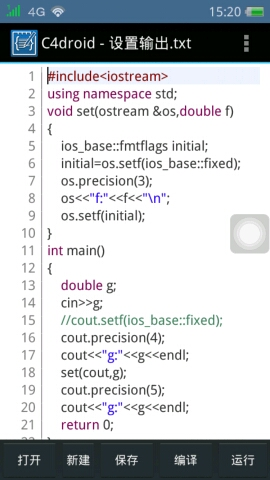os.setf(initial);这句到底起什么作用？

os.setf(initial);不管我有没有注释掉这条语句，运行结果都一样，感觉这条语句好像是多余的。4个回答

// using ios_base::fmtflags
#include // std::cout, std::ios_base, std::ios,
// std::hex, std::showbase
int main () {

// using fmtflags as class member constants:
std::cout.setf (std::ios_base::hex , std::ios_base::basefield);
std::cout.setf (std::ios_base::showbase);
std::cout << 100 << '\n';

// using fmtflags as inherited class member constants:
std::cout.setf (std::ios::hex , std::ios::basefield);
std::cout.setf (std::ios::showbase);
std::cout << 100 << '\n';

// using fmtflags as object member constants:
std::cout.setf (std::cout.hex , std::cout.basefield);
std::cout.setf (std::cout.showbase);
std::cout << 100 << '\n';

// using fmtflags as a type:
std::ios_base::fmtflags ff;
ff = std::cout.flags();
ff &= ~std::cout.basefield; // unset basefield bits
ff |= std::cout.hex; // set hex
ff |= std::cout.showbase; // set showbase
std::cout.flags(ff);
std::cout << 100 << '\n';

// not using fmtflags, but using manipulators:
std::cout << std::hex << std::showbase << 100 << '\n';

return 0;
}

c++库里面的东西，是一个标志类型的变量。有用的，仅仅是当前你用不到